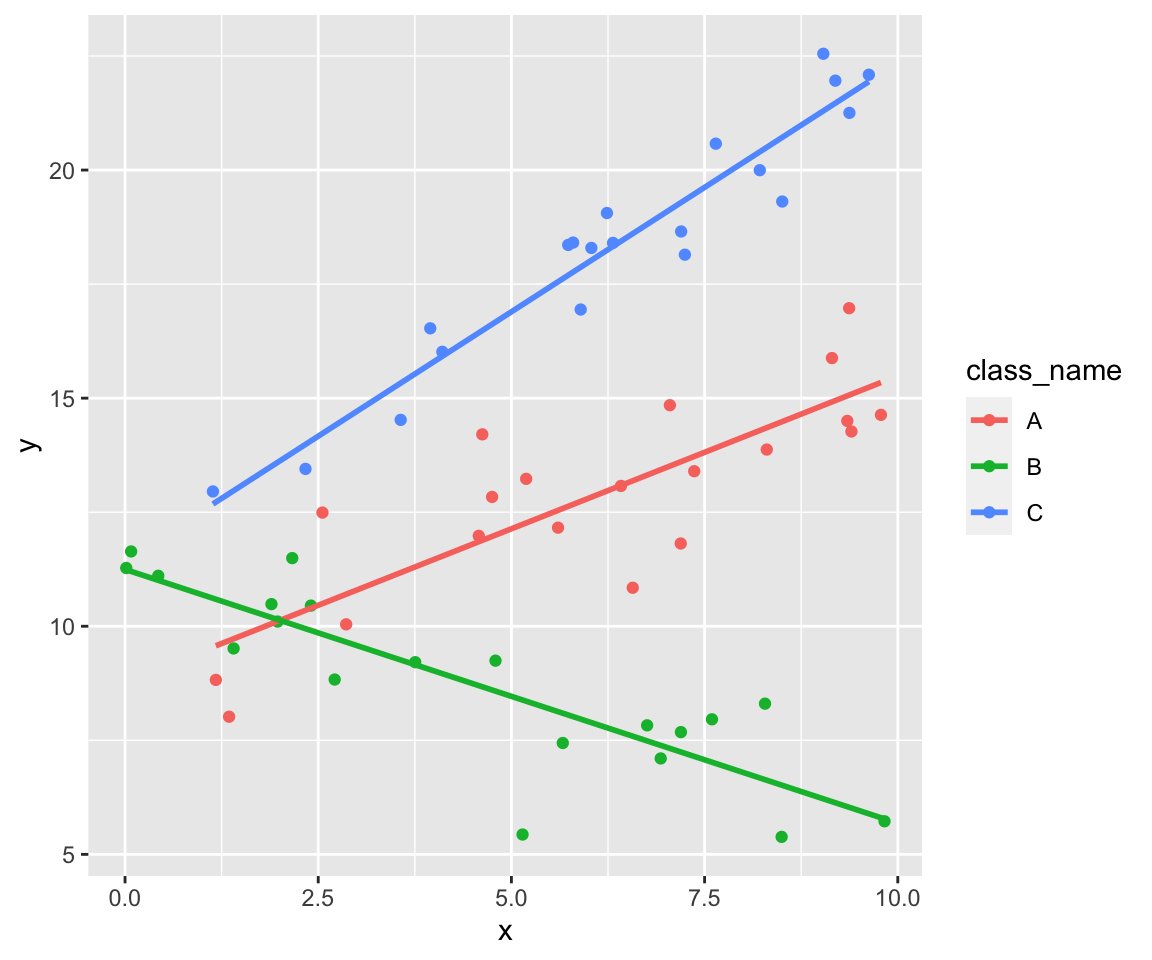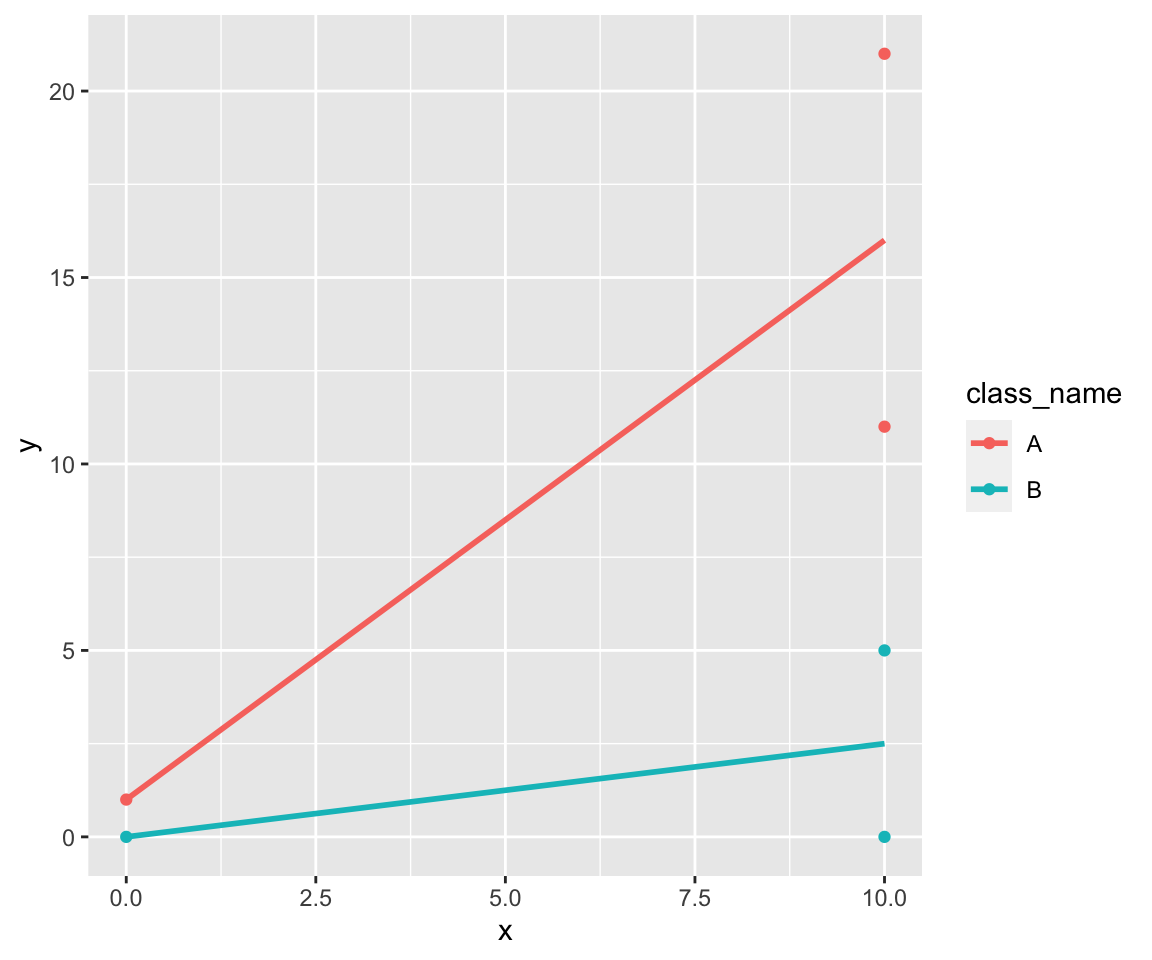Last Thursday I watched Wolfgang Viechtbauer’s stream on Twitch. Wolfgang spoke about linear regression. (If you’re interested in the results: Wolfgang lists the R-code he wrote during the stream at https://www.wvbauer.com/doku.php/live_streams.)

During the stream we had an example with one categorical and one numeric predictor and we built a model with interaction between these two predictors. So the result is a straight line for each value of the categorival group.

The question was if it makes a difference if you build a simple linear model for each group.

So let’s look at it.

## Example

Let’s start and build some sample data:

  1 2 3 4 5 6 7 8 9 10 11 12 13 14 15 16 17 18 19 20 21 22 23  options(tidyverse.quiet = TRUE) library(tidyverse) parameters <- tribble( ~class_name, ~intercept, ~slope, ~sd, "A", 5, 0.5, 5, "B", 6, -0.5, 5, "C", 7, 1, 5, ) generate_class <- function(n, class_name, intercept, slope, sd) { start <- 0 end <- 10 values <- tibble( class_name = rep(class_name, times = n), x = runif(n, start, end), y = intercept + x * slope + rnorm(n, sd) ) return(values) } set.seed(42) data <- pmap_dfr(parameters %>% mutate(n = 20), generate_class) 

So we get three clouds of points. Each cloud follows its own line.

 1 2 3 4  data %>% ggplot(aes(x, y, color = class_name)) + geom_point() + stat_smooth(formula = y ~ x, method = lm, se = FALSE)### Build one linear model

We’re building the model without an intercept by using 0 + as start of the formula so we get the intercept for each group directly (without building the sum of the reference group and the other groups.)

 1 2  model_complete <- lm(y ~ 0 + class_name + class_name:x, data = data) summary(model_complete) 
  1 2 3 4 5 6 7 8 9 10 11 12 13 14 15 16 17 18 19 20 21 22  ## ## Call: ## lm(formula = y ~ 0 + class_name + class_name:x, data = data) ## ## Residuals: ## Min 1Q Median 3Q Max ## -2.94880 -0.65484 0.06566 0.66447 2.32326 ## ## Coefficients: ## Estimate Std. Error t value Pr(>|t|) ## class_nameA 8.78346 0.60220 14.586 < 2e-16 *** ## class_nameB 11.24628 0.42291 26.592 < 2e-16 *** ## class_nameC 11.43872 0.69364 16.491 < 2e-16 *** ## class_nameA:x 0.67056 0.09012 7.441 7.93e-10 *** ## class_nameB:x -0.55649 0.07967 -6.985 4.35e-09 *** ## class_nameC:x 1.09090 0.10240 10.653 6.95e-15 *** ## --- ## Signif. codes: 0 '***' 0.001 '**' 0.01 '*' 0.05 '.' 0.1 ' ' 1 ## ## Residual standard error: 1.071 on 54 degrees of freedom ## Multiple R-squared: 0.9948, Adjusted R-squared: 0.9942 ## F-statistic: 1727 on 6 and 54 DF, p-value: < 2.2e-16 

### Build three different models

Now build a linear model for each group.

 1 2 3 4 5  model_split <- data %>% group_by(class_name) %>% group_map(~ coef(summary(lm(y ~ x, data = .x)))) model_split 
  1 2 3 4 5 6 7 8 9 10 11 12 13 14  ## [] ## Estimate Std. Error t value Pr(>|t|) ## (Intercept) 8.7834572 0.7482108 11.739282 7.181449e-10 ## x 0.6705587 0.1119703 5.988718 1.153349e-05 ## ## [] ## Estimate Std. Error t value Pr(>|t|) ## (Intercept) 11.2462761 0.40929811 27.476980 3.773904e-16 ## x -0.5564857 0.07710095 -7.217624 1.027562e-06 ## ## [] ## Estimate Std. Error t value Pr(>|t|) ## (Intercept) 11.438716 0.5000135 22.87681 9.354783e-15 ## x 1.090904 0.0738192 14.77805 1.653770e-11 

### Comparison

As you can see the coefficients are the same but the standard error of the coefficients are different.

“What’s the reason?” I was asking myself.

## Simple example

So I began to look at a simpler example:

  1 2 3 4 5 6 7 8 9 10 11  data <- tribble( ~class_name, ~x, ~y, "A", 0, 1, "A", 10, 11, "A", 10, 21, "B", 0, 0, "B", 10, 0, "B", 10, 5, ) data 
 1 2 3 4 5 6 7 8 9  ## # A tibble: 6 x 3 ## class_name x y ## ## 1 A 0 1 ## 2 A 10 11 ## 3 A 10 21 ## 4 B 0 0 ## 5 B 10 0 ## 6 B 10 5 
 1 2 3 4  data %>% ggplot(aes(x, y, color = class_name)) + geom_point() + stat_smooth(formula = y ~ x, method = lm, se = FALSE)So we’ve only two groups. The linear model is simple:

 1  lm(y ~ class_name * x, data) %>% summary() 
  1 2 3 4 5 6 7 8 9 10 11 12 13 14 15 16 17 18  ## ## Call: ## lm(formula = y ~ class_name * x, data = data) ## ## Residuals: ## 1 2 3 4 5 6 ## -4.441e-16 -5.000e+00 5.000e+00 1.648e-15 -2.500e+00 2.500e+00 ## ## Coefficients: ## Estimate Std. Error t value Pr(>|t|) ## (Intercept) 1.0000 5.5902 0.179 0.875 ## class_nameB -1.0000 7.9057 -0.126 0.911 ## x 1.5000 0.6847 2.191 0.160 ## class_nameB:x -1.2500 0.9682 -1.291 0.326 ## ## Residual standard error: 5.59 on 2 degrees of freedom ## Multiple R-squared: 0.8201, Adjusted R-squared: 0.5501 ## F-statistic: 3.038 on 3 and 2 DF, p-value: 0.2574 

### Step by Step computation

But how can we compute the linear model step by step?

#### The data

First let’s split the data into $y$ and $X$:

  1 2 3 4 5 6 7 8 9 10 11 12  y <- data %>% pull(y) X <- data %>% mutate( x0 = 1, x1 = class_name != "A", x2 = x, x3 = x1*x ) %>% select(x0, x1, x2, x3) %>% as.matrix() X 
 1 2 3 4 5 6 7  ## x0 x1 x2 x3 ## [1,] 1 0 0 0 ## [2,] 1 0 10 0 ## [3,] 1 0 10 0 ## [4,] 1 1 0 0 ## [5,] 1 1 10 10 ## [6,] 1 1 10 10 
 1  y 
 1  ##  1 11 21 0 0 5 

So $y$ contains the values of the dependent variable. $X$ is a matrix with $1$ in the first column called $x_0$. $x_1 .. x_3$ are the predictors. $x_3$ is the interaction between $x_1$ and $x_2$.

#### The model

Now we can write our model as:

$$y = X \beta + \epsilon$$ We’re searching for $b$ so that $\hat{y} = X b$ is the prediction which minimizes the sum of the squared residuals.

We get $b$ as

$$b = (X^t X)^{-1} X^t y$$

So let’s compute it: (solve computes the inverse of a matrix)

 1 2 3 4  Xt <- t(X) b <- solve(Xt %*% X) %*% Xt %*% y round(b, 3) 
 1 2 3 4 5  ## [,1] ## x0 1.00 ## x1 -1.00 ## x2 1.50 ## x3 -1.25 

#### Standard Errors of the coefficients

Now we’re looking at the standard errors of the coefficients:

We can compute them by

$$Cov(b) = \sigma^2 (X^t X)^{-1}$$

A predictior for $\sigma^2$ is the mean squared error: $$s^2 = \frac{\sum{(y_i - \hat{y})^2}}{df}$$ In our case the degrees of freedom are $df = n - 4$.

 1 2 3 4  yh <- X %*% b df <- length(y) - 4 MSE <- sum((y - yh)**2) / df MSE 
 1  ##  31.25 

So the standard errors of the coefficients are the main diagonal of this matrix:

 1  (solve(Xt %*% X) * MSE) %>% sqrt() 
 1  ## Warning in sqrt(.): NaNs produced 
 1 2 3 4 5  ## x0 x1 x2 x3 ## x0 5.590170 NaN NaN 1.7677670 ## x1 NaN 7.905694 1.7677670 NaN ## x2 NaN 1.767767 0.6846532 NaN ## x3 1.767767 NaN NaN 0.9682458 

These are the same as computed by lm:

 1  lm(y ~ class_name * x, data) %>% summary() 
  1 2 3 4 5 6 7 8 9 10 11 12 13 14 15 16 17 18  ## ## Call: ## lm(formula = y ~ class_name * x, data = data) ## ## Residuals: ## 1 2 3 4 5 6 ## -4.441e-16 -5.000e+00 5.000e+00 1.648e-15 -2.500e+00 2.500e+00 ## ## Coefficients: ## Estimate Std. Error t value Pr(>|t|) ## (Intercept) 1.0000 5.5902 0.179 0.875 ## class_nameB -1.0000 7.9057 -0.126 0.911 ## x 1.5000 0.6847 2.191 0.160 ## class_nameB:x -1.2500 0.9682 -1.291 0.326 ## ## Residual standard error: 5.59 on 2 degrees of freedom ## Multiple R-squared: 0.8201, Adjusted R-squared: 0.5501 ## F-statistic: 3.038 on 3 and 2 DF, p-value: 0.2574 

As you can see the covariance matrix isn’t diagnal. Points from “the other group” also effect the standard error.

So the errors are different to the errors of two independent models:

 1 2 3  data %>% group_by(class_name) %>% group_map(~ coef(summary(lm(y ~ x, data = .x)))) 
 1 2 3 4 5 6 7 8 9  ## [] ## Estimate Std. Error t value Pr(>|t|) ## (Intercept) 1.0 7.0710678 0.1414214 0.9105615 ## x 1.5 0.8660254 1.7320508 0.3333333 ## ## [] ## Estimate Std. Error t value Pr(>|t|) ## (Intercept) 2.56395e-16 3.5355339 7.251946e-17 1.0000000 ## x 2.50000e-01 0.4330127 5.773503e-01 0.6666667 

### Separate Models

For completeness let’s check the manual way for one single model here:

  1 2 3 4 5 6 7 8 9 10 11 12 13 14 15  data_single <-data %>% filter(class_name == "A") y <- data_single %>% pull(y) X <- data_single %>% mutate( x0 = 1, x1 = class_name != "A", x2 = x, x3 = x1*x ) %>% select(x0, x2) %>% as.matrix() X 
 1 2 3 4  ## x0 x2 ## [1,] 1 0 ## [2,] 1 10 ## [3,] 1 10 
 1  y 
 1  ##  1 11 21 
 1 2 3 4  Xt <- t(X) b <- solve(Xt %*% X) %*% Xt %*% y round(b, 3) 
 1 2 3  ## [,1] ## x0 1.0 ## x2 1.5 
 1 2 3 4  yh <- X %*% b df <- length(y) - 2 MSE <- sum((y - yh)**2) / df MSE 
 1  ##  50 
 1  (solve(Xt %*% X) * MSE) %>% sqrt() 
 1  ## Warning in sqrt(.): NaNs produced 
 1 2 3  ## x0 x2 ## x0 7.071068 NaN ## x2 NaN 0.8660254